# KSEEB Solutions for Class 7 Maths Chapter 8 Comparing Quantities Ex 8.3

Students can Download Chapter 8 Comparing Quantities Ex 8.3, Question and Answers, Notes Pdf, KSEEB Solutions for Class 7 Maths, Karnataka State Board Solutions help you to revise complete Syllabus and score more marks in your examinations.

## Karnataka State Syllabus Class 7 Maths Chapter 8 Comparing Quantities Ex 8.3

Question 1.
Tell what is the profit or loss in the following transactions. Also find profit per cent or loss per cent in each case.
a) Gardening shears bought for ₹ 250 and sold for ₹ 325.
Solution:
C. P = Rs 250
S. P = Rs. 325
S.P > C.P
∴ It is profit.
Total profit = S.P – C.P
= 325 – 250 = Rs 75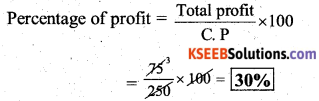b) A refrigerator bought for ₹ 12,000 and sold at ₹ 13,500.
Solution:
C. P = Rs. 12,000
S.P = Rs. 13,500
S.P > C.P
It is profit
Total profit = S. P – C. P
= 13,500 – 12,000
= Rs.1,500/-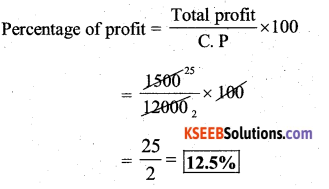c) A cupboard bought for ₹ 2,500 and sold at ₹ 3,000.
Solution:
C.P = Rs. 2,500
S. P = Rs. 3,000
S.P > C.P
It is profit
Total profit = S. P – C. P
= 3000 – 2500
= Rs. 500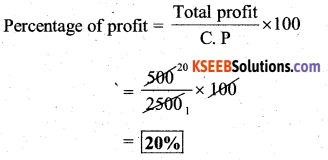d) A skirt bought for 250 and sold at ₹ 150.
C.P = Rs. 250/-
S.P = Rs. 150/-
S.P < C.P
∴ It is loss
Total loss = C.P – S.P
= 250 – 150 = Rs. 100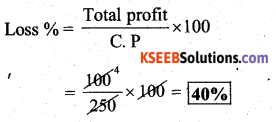Question 2.
Convert each part of the ratio to percentage :
a) 3 : 1
Solution:
Total parts = 3 + 1 = 4
Percentage of First part of the ratio =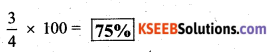Percentage of 2nd part of the ration =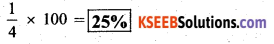b) 2 : 3 : 5
Solution:
Total parts = 2 + 3 + 5 = 10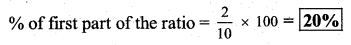% of second part of the ratio =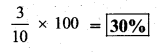% of third part of the ratio =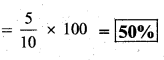c) 1 : 4
Total parts = 1 + 4 = 5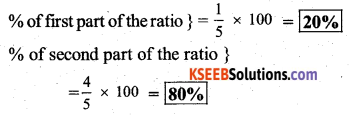d) 1 : 2 : 5
Total parts. = 1 + 2 + 5 = 8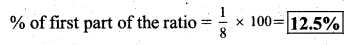% of second part of the ratio =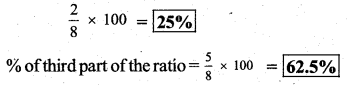Question 3.
The population of a city decreased from 25,000 to 24,500. Find the percentage decrease.
Solution:
Decreased population = 25,000 – 24,500
= 500
Percentage of decrease =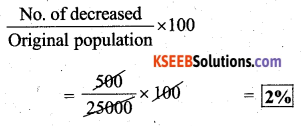Question 4.
Arun bought a car for 3, 50, 000. The next year, the price went upto ₹ 3,70,000. What was the percentage of price increase ?
Solution:
Price of a car = Rs. 3,50,000
Increased price of a car = Rs. 3,70,000
Total increase = Rs. 20,000
Percentage of price increase =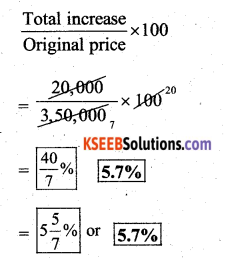Question 5.
I buy a T. V. for ₹ 10,000 and sell it at a profit of 20%. How much money do I get for it ?
Solution:
C.P of a T.V = Rs. 10,000
Profit = 20%
20% of Rs. 10,000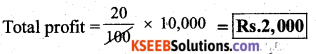∴ Amount I get = C. P + profit
= 10,000 + 2,000
= Rs. 12,000Question 6.
Juhi sells a washing machine for ₹ 13,500. She loses 20% in the bargain. What was the price at which she bought it ?
Solution:
S. P = C. P – Loss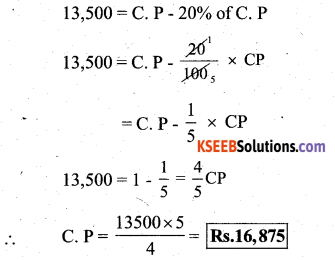∴ She bought that washing machine for Rs. 16,875.

Question 7.
i) Chalk contains calcium, carbon and oxygen in the ratio 10 : 3 : 12. Find the percentage of carbon in chalk.
Solution:
Total parts = 10 + 3 + 12 = 25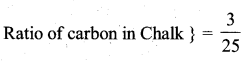% of carbon in chalk =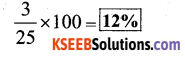ii) If in a stick of chalk. carbon is 3g, what is the chalk stick?
Solution:
Let the weight of chalk stick be ‘M’
Then 12% of M = 3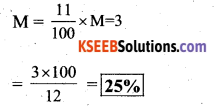∴ The weight of the chalk stick is 25 grams.

Question 8.
Amina buys a book for ₹ 275 and sells it at a loss of 15%. How much does she sell it for ?
Solution:
C. P of a book = Rs. 275
loss = 15% of C. P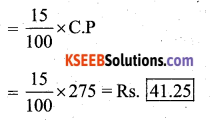S.P = C.P – Loss
= 275 – 41.25
= Rs. 233.751
∴ She sells it for Rs. 233.75.Question 9.
Find the amount to be paid at the end of 3 years in each case:
a) Principal = ₹ 1,200 at 12 % p.a
P = Rs. 1200/-
R = 12%
T = 3 years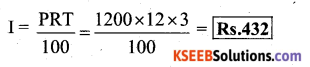A = P + I = 1200 + 432 = Rs. 1,632
∴ The amount to paid at the end of 3 years is Rs. 1,632

b) Principal = ₹ 7,500 at 5% p.a
P = Rs. 7,500
R = 5%
T = 3 years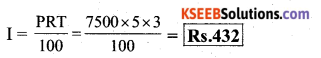A = P + I
= 7500 + 1125
= Rs. 8,625
∴ The amount to be paid at the end of 3 years is Rs. 8,625.

Question 10.
What rate gives ₹ 280 as interest on a sum of ₹ 56,000 in 2 years ?
Solution:
P = Rs. 56,000/-
R = ?
T = 2 years
I = Rs. 280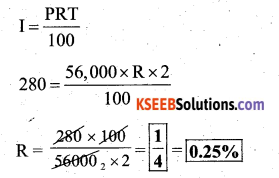∴ The rate of interest is 0.25% per annum.Question 11.
If Meena gives an interest of ? 45 for one year at 9% rate p.a. What is the sum she has borrowed ?
Solution:
P = ?
R = 9%
T = 1 years
I = Rs. 45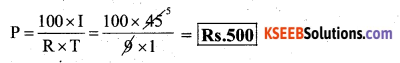∴ She has borrowed the sum of Rs. 500